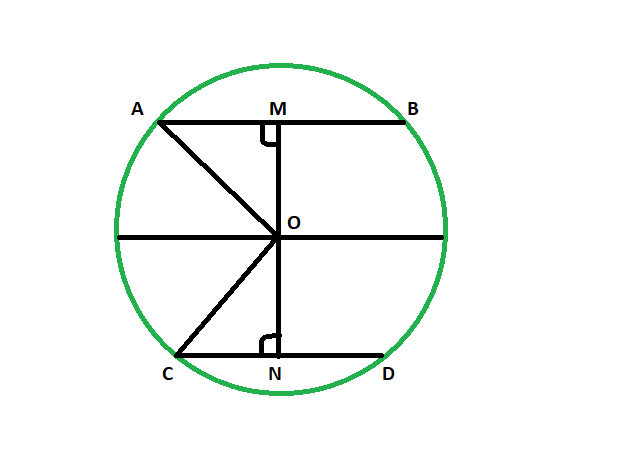Related Articles
Distance of chord from center when distance between center and another equal length chord is given
• Last Updated : 09 Mar, 2021

Given two equal length chords of a circle and Distance between the centre and one chord. The task is here to find the distance between the centre and the other chord.
Examples:

```Input: 48
Output: 48

Input: 82
Output: 82```Below is the implementation of the above approach:
Approach
Let AB & CD be the two equal chords of the circle having center at O.OM be the given distance of the chord AB from center.
now in triangle AOM and CON
OA = OC (radii of same circle)
MA = CN (since OM and ON are the perpendicular to the chord and it bisects the chord and AM = MB & CN = CD)
angle AMO = angle ONC = 90 degrees
so the triangles are congruent
so, OM = ON

Equal chords of a circle are equidistant from the centre of a circle.

Below is the implementation of the above approach:

## C++

 `// C++ program to find the distance of chord``// from center when distance between center``// and another equal length chord is given` `#include ``using` `namespace` `std;` `void` `lengequichord(``int` `z)``{``    ``cout << ``"The distance between the "``         ``<< ``"chord and the center is "``         ``<< z << endl;``}` `// Driver code``int` `main()``{``    ``int` `z = 48;``    ``lengequichord(z);``    ``return` `0;``}`

## Java

 `// Java program to find the distance of chord``// from center when distance between center``// and another equal length chord is given/` `import` `java.io.*;` `class` `GFG``{``    ` `static` `void` `lengequichord(``int` `z)``{``    ``System.out.println (``"The distance between the "``+``    ``"chord and the center is "``+ z );``}` `// Driver code``public` `static` `void` `main (String[] args)``{` `    ``int` `z = ``48``;``    ``lengequichord(z);``}``}` `// This code is contributed by jit_t.`

## Python 3

 `# Python 3 program to find the distance of chord``# from center when distance between center``# and another equal length chord is given` `def` `lengequichord(z):``    ``print``(``"The distance between the"` `,``          ``"chord and the center is"` `, z )` `# Driver code``if` `__name__ ``=``=` `"__main__"``:``    ``z ``=` `48``    ``lengequichord(z)` `# This code is contributed``# by ChitraNayal`

## C#

 `// C# program to find the distance of chord``// from center when distance between center``// and another equal length chord is given``using` `System;` `class` `GFG``{``    ` `    ``static` `void` `lengequichord(``int` `z)``    ``{``        ``Console.WriteLine(``"The distance between the "``+``        ``"chord and the center is "``+ z );``    ``}``    ` `    ``// Driver code``    ``public` `static` `void` `Main ()``    ``{``    ` `        ``int` `z = 48;``        ``lengequichord(z);``    ``}``}` `// This code is contributed by AnkitRai01`

## Javascript

 ``
Output:
`The distance between the chord and the center is 48`

Attention reader! Don’t stop learning now. Get hold of all the important DSA concepts with the DSA Self Paced Course at a student-friendly price and become industry ready.

My Personal Notes arrow_drop_up